Definitions of Square Dance Calls and Concepts

Index -->  Plus  |  A1  |  A2  |  C1  |  C2  |  C3A  |  C3B  |  C4  |  NOL  |
Definitions (Text Only) -->  Plus  |  A1  |  A2  |  C1  |  C2  |  C3A  |  C3B  |  C4  |  NOL  |
 Find call:

Tandem \$B\$N?M(B, \$B8~\$+\$\$9g\$C\$??M(B, \$B\$^\$?\$OGXCf9g\$o\$;\$N?M\$+\$i(B.

Leader \$B\$,1&\$X(B Zing (1/4 Right and Run) \$B\$r\$9\$k4V\$K(B, Trailer \$B\$O(B Circulate \$B\$r\$7\$FM?\$(\$i\$l\$?(B direction \$B\$NJ}\$X(B 1/4 \$B8~\$-\$rJQ\$(\$k\$+(B, anything \$B\$N%3!<%k\$r9T\$\$\$^\$9(B.

Pitch direction \$B\$O(B, \$B%+%C%W%k\$^\$?\$O(B Mini-Wave \$B\$G=*\$o\$j\$^\$9(B.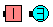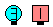Pitch Left\$B\$NA0(B \$B8e(B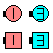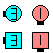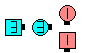Pitch Single Wheel\$B\$NA0(B Leaders Right Zing as Trailers Circulate\$B\$N8e(B original Trailers Single Wheel\$B\$N8e(B (\$B=*\$o\$j(B)

\$BCm
• \$B\$b\$7(B direction \$B\$d(B anything call \$B\$,\$J\$+\$C\$?\$H\$-\$O(B, Trailer \$B\$O(B Circulate \$B\$N8e(B, \$B2?\$b\$7\$^\$;\$s(B.
• \$B\$b\$7(B 'Pitch' \$B\$N8@MU\$NA0\$K(B direction \$B\$,\$"\$k\$H\$-(B, Leader \$B\$O(B Zing \$B\$r(B, \$B\$=\$N(B direction \$B\$NJ}\$X9T\$\$\$^\$9(B (\$B\$9\$J\$o\$A(B, 'Left Pitch Right' \$B\$G\$O(B Leader \$B\$O(B Left Zing \$B\$r\$7(B, Trailer \$B\$O(B Circulate and 1/4 Right \$B\$r\$7\$^\$9(B).
• \$B\$b\$7M?\$(\$i\$l\$?(B direction \$B\$,(B 'In' \$B\$^\$?\$O(B 'Out' \$B\$N\$H\$-\$O(B, 1/4 In | Out \$B\$r\$7\$^\$9(B (Face In | Out \$B\$G\$O\$J\$/(B).
• Pitch Right \$B\$O(B Latch On [C3A] \$B\$HF1\$8\$G\$9(B.
•Comments? Questions? Suggestions?

https://www.ceder.net/def/pitch.php?language=japan&level=master
13-August-2020 09:36:11